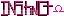-=+=- -=+=- -=+=- -=+=- -=+=- -=+=- -=+=- -=+=- -=+=- -=+=- -=+=- -=+=- -=+=- -=+=- -=+=- -=+=- -=+=- -=+=- -=+=- -=+=- -=+=- -=+=- -=+=- -=+=- -=+=- -=+=- -=+=- -=+=- -=+=- -=+=- (c) WidthPadding Industries 1987 0|151|0 -=+=- -=+=- -=+=- -=+=- -=+=- -=+=- -=+=- -=+=- -=+=- -=+=- -=+=- -=+=- -=+=- -=+=- -=+=- -=+=- -=+=- -=+=- -=+=- -=+=- -=+=- -=+=- -=+=- -=+=- -=+=- -=+=- -=+=- -=+=- -=+=- -=+=- Socoder -> Question of the Day -> is pong un-cool Wed, 29 Apr 2009, 03:47 cthugI realised the other day I have never made a pong clone, so I am thinking of making one. So is making a pong clone un-cool or newbie. -=-=- CTHUG.co.nr Get UbuntuWed, 29 Apr 2009, 04:34 JayenkaiLike a plain Space Invaders, if you just do it as a bog-standard version, it's not exactly the most exciting thing to play anymore! Add something new to the mix, and even pong can be interesting.. -=-=- ''Load, Next List!''Wed, 29 Apr 2009, 04:40 cthugyeah, you can write pong in 100 lines of code or a million; add somthing a little different.-=-=- CTHUG.co.nr Get UbuntuWed, 29 Apr 2009, 04:58 JL235It depends entirely on the Pong. A few weeks ago there was an awesome single-player side-scrolling pong clone on Co-Op. It was bouncing balls rather then shooting enemies, but it was essentially pong. If you make a really dull pong clone, then I'd say it's a very simple straight forward project. No matter how simple I wouldn't say it's un-cool or newbie. Wed, 29 Apr 2009, 06:01 Afr0I made a Pong clone a while ago called 'Bullet Pong'. Check it out if you want inspiration. -=-=- Afr0 Games Project Dollhouse on Github - Please fork!Wed, 29 Apr 2009, 07:32 9572ADPong must be cool, otherwise why would there be six of them in the XBox Live Community Games already? -=-=- All the raw, animal magnetism of a rutabaga.Wed, 29 Apr 2009, 11:32 SchererererPong is ALWAYS cool. no doubt about it.Wed, 29 Apr 2009, 15:02 JayenkaiImpromptu Question of the Day : How would YOU make Pong better? -=-=- ''Load, Next List!''Fri, 01 May 2009, 08:41 PioMaybe Pang Pong: the ball gets a little bigger all the time, and when it gets big enough, any player can split it in two in Pang way, by shooting a spear through it. Any ball not returned counts +1 for the opposite player, but action doesn't stop until all balls are gone. Or: Just add the ability to add a spin to the ball and to hit it harder.